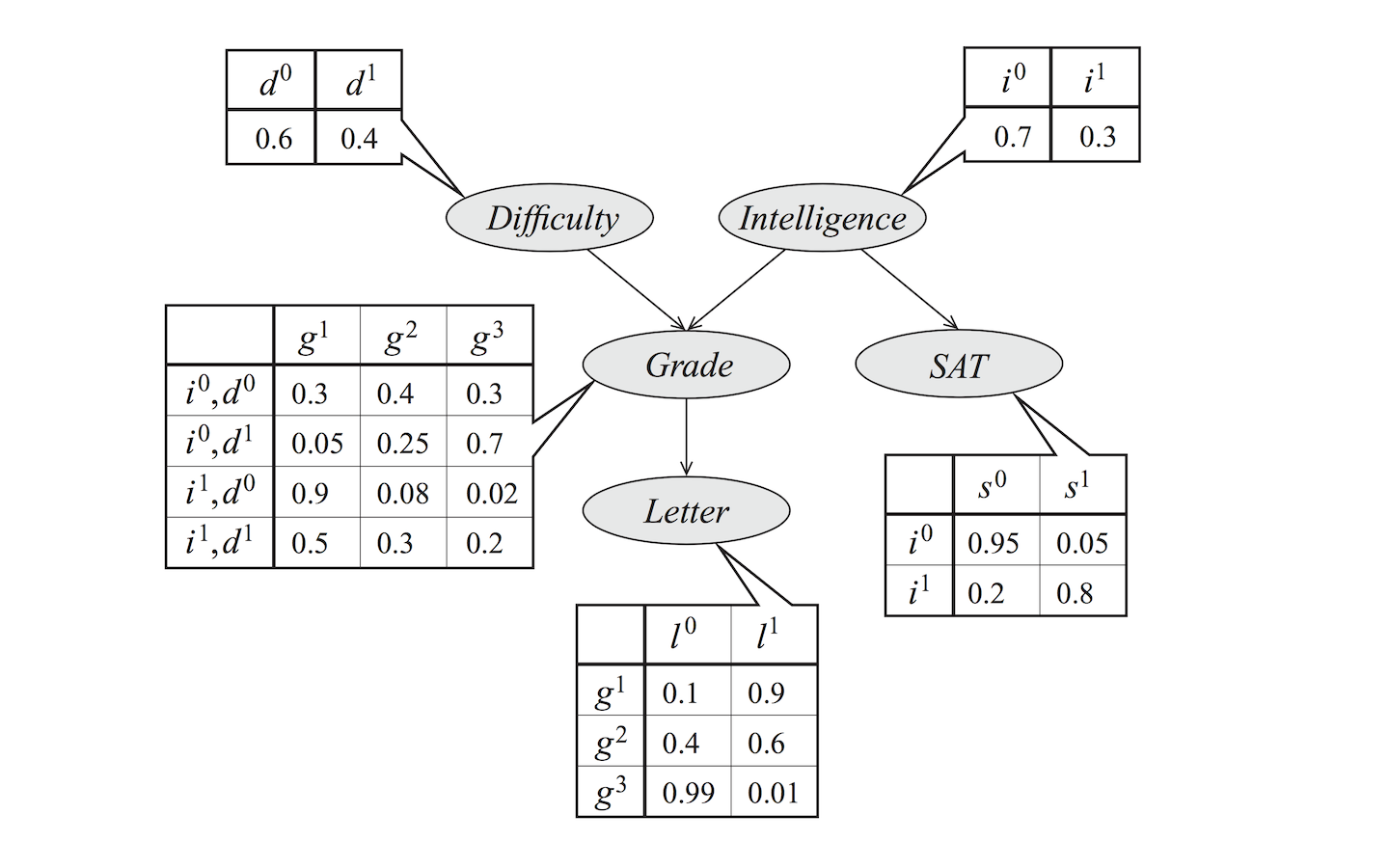# Bayesian Network

## Reprenstation of Directed Graph — Bayesian Network

Posted by Chaway on 2017-03-21

## Overview：

PGM（i.e. Probabilistic Graphical Model）简单来说，可以看成由图和局部条件概率分布组合而成。图中的每个节点 $$V_i$$ 表示一个随机变量，相邻的节点在图中用边 $$E_i$$ 连接。Figure 1:Student Bayesian Network with CPDs

## Probablity Distribution & Factorization：

$P(D,I,G,S,L) = P(I)P(D|I)P(G|D,I)P(L|G,D,I)P(S|L,G,D,I)$

$P(D,I,G,S,L) = P(D)P(I)P(S|I)P(G|I,D)P(L|G)$

$P(X_1,X_2,X_3,\cdots,X_n) = \prod_i P(X_i|Parents(X_i))$

## Independency：

#### 网络结构中的独立性：

##### 2.全局马尔可夫独立性：

• 因果迹 (Causal Trail): $$X \rightarrow Z \rightarrow Y$$ ，当且仅当 Z 没有被观察时有效(active)
• 证据迹 (Evidential Trail): $$X \leftarrow Z \leftarrow Y$$ ，当且仅当 Z 没被观察时有效
• 共同的原因 (Common Cause): $$X \leftarrow Z \rightarrow Y$$ ， 当且仅当 Z 没有被观察时有效
• 共同的作用 (Common Effect): $$X \rightarrow Z \leftarrow Y$$ (v-structure) ， 当且仅当 Z 被观察时有效

• 迹中包含的 v-结构 ，$$X_{i-1} \rightarrow X_i \leftarrow X_{i+1}$$，则 $$X_i$$ 或其一个后代在 Z 中.
• 迹上其他节点都不在 Z 中（包括两个端节点）。

$$Z$$ 已知的条件下，如果 $$X$$$$Y$$之间没有有效迹相连，则称 $$X$$$$Y$$ 是 d-分离(d-separation)的

## I-map：

$$K$$ 是与独立性集合 $$I(K)$$ 相关联的任意一个图对象。如果对独立性集合 $$I$$$$I(K) \subseteq I$$ 则称 $$K$$ 是一个 I-map。

• 概率分布 P 满足与图 G 相关的局部独立性的充要条件是 P 可以在 G 上进行分解
• P 满足 G 中的局部独立性的充要条件是 P 满足 d-分离准则的全局独立性